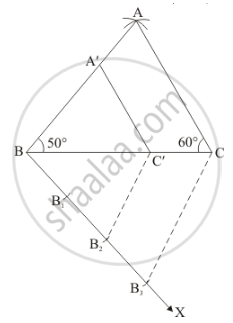Share

# Construct a Triangle Similar to a Given δAbc Such that Each of Its Sides is (2/3)Rd of the Corresponding Sides of δAbc. It is Given that Bc = 6 Cm, ∠B = 50° and ∠C = 60°. - CBSE Class 10 - Mathematics

#### Question

Construct a triangle similar to a given ΔABC such that each of its sides is (2/3)rd of the corresponding sides of ΔABC. It is given that BC = 6 cm, ∠B = 50° and ∠C = 60°.

#### Solution

Given that

Construct a triangle of given data, BC = 6 cm, ∠B = 50° and ∠C = 60° and then a triangle similar to it whose sides are (2/3)rd of the corresponding sides of ΔABC.

We follow the following steps to construct the givenStep of construction

Step: I- First of all we draw a line segment Bc = 60°.

Step: II- With as centre draw an angle ∠B = 50°.

Step: III- With as centre draw an angle ∠C = 60° which intersecting the line drawn in step II at A.

Step: IV- Joins AB and Ato obtain ΔABC.

Step: V -Below BC, makes an acute angle ∠CBX = 60°.

Step: VI -Along BX, mark off three points B1, B2 and B3 such that BB1 = B1B2 = B2B3

Step: VII -Join B3C.

Step: VIII -Since we have to construct a triangle each of whose sides is two-third of the corresponding sides of ΔABC.

So, we take two parts out of three equal parts on BX from point B2 draw B2C' || B3C, and meeting BC at C’.

Step: IX -From C’ draw C'A' || AC and meeting AB at A

Thus, ΔA'BC' is the required triangle, each of whose sides is two third of the corresponding sides of ΔABC.

Is there an error in this question or solution?

#### APPEARS IN

Solution Construct a Triangle Similar to a Given δAbc Such that Each of Its Sides is (2/3)Rd of the Corresponding Sides of δAbc. It is Given that Bc = 6 Cm, ∠B = 50° and ∠C = 60°. Concept: Division of a Line Segment.
S